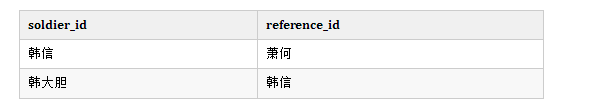# 递归最佳解析

0收藏f(n)=f(n-1) + 1, 存在 f(1) = 1

f(n) 表示自己的数字，f(n - 1) 表示前面一个人的报数，f(1) 表示第一个人知道自己是第一个报的数字。

``````public int f(int n) {
if (n == 1) return 1;
return f(n-1) + 1;
}``````

1.一个问题可以分解多个子问题

2.问题本身与分解后的子问题，除了数据规模不同，求解算法相同

『求解自己的报数』和前面一个人『求解自己的报数』思路是一模一样。

3.存在递归终止条件

``````f(n) = f(n-1) + f(n-2)
``````

``````f(1) = 1;
f(2) = 2;
f(n) = f(n-1) + f(n-2);``````

``````public int f(n) {
if(n == 1) return 1;
if(n ==2) return 2;
return f(n-1) + f(n-2);
}``````

``````Exception in thread "main" java.lang.StackOverflowError
``````

``````public int f(int n) {
if (n == 1) return 1;
if (n == 2) return 2;

// hasSolvedList 可以理解成一个 Map，key 是 n，value 是 f(n)
if (hasSolvedMap.containsKey(n)) {
return hasSovledMap.get(n);
}

int ret = f(n-1) + f(n-2);
hasSovledMap.put(n, ret);
return ret;
}``````

f(n) = f(n-1) +1;

``````public int f(n) {
int r = 1;
for(int i = 2; i <= n; i++) {
r += 1;
}
return r;
}``````

``````public int f(int n) {
if (n == 1) return 1;
if (n == 2) return 2;

int ret = 0;
int pre = 2;
int prepre = 1;
for (int i = 3; i <= n; ++i) {
ret = pre + prepre;
prepre = pre;
pre = ret;
}
return ret;
}``````

``````public int findRootReferId(int soldierId) {
Integer referId = "select reference_id from [table] where soldier_id = soldierId";
if (referId == null) return soldierId;
return findRootReferId(referId);
}``````相关问题
社区精华内容